Search by Topic

Resources tagged with Maths Supporting SET similar to Flight Path:

Filter by: Content type:
Age range:
Challenge level:

There are 89 results

Broad Topics > Applications > Maths Supporting SETFlight Path

Age 16 to 18 Challenge Level:

Use simple trigonometry to calculate the distance along the flight path from London to Sydney.Spherical Triangles on Very Big Spheres

Age 16 to 18 Challenge Level:

Shows that Pythagoras for Spherical Triangles reduces to Pythagoras's Theorem in the plane when the triangles are small relative to the radius of the sphere.Air Routes

Age 16 to 18 Challenge Level:

Find the distance of the shortest air route at an altitude of 6000 metres between London and Cape Town given the latitudes and longitudes. A simple application of scalar products of vectors.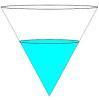Fill Me up Too

Age 14 to 16 Challenge Level:

In Fill Me Up we invited you to sketch graphs as vessels are filled with water. Can you work out the equations of the graphs?Witch's Hat

Age 11 to 16 Challenge Level:

What shapes should Elly cut out to make a witch's hat? How can she make a taller hat?Immersion

Age 14 to 16 Challenge Level:

Various solids are lowered into a beaker of water. How does the water level rise in each case?Speed-time Problems at the Olympics

Age 14 to 16 Challenge Level:

Have you ever wondered what it would be like to race against Usain Bolt?Far Horizon

Age 14 to 16 Challenge Level:

An observer is on top of a lighthouse. How far from the foot of the lighthouse is the horizon that the observer can see?Age 14 to 18 Challenge Level:

How would you design the tiering of seats in a stadium so that all spectators have a good view?Whose Line Graph Is it Anyway?

Age 16 to 18 Challenge Level:

Which line graph, equations and physical processes go together?The Wrong Stats

Age 16 to 18 Challenge Level:

Why MUST these statistical statements probably be at least a little bit wrong?Polygon Walk

Age 16 to 18 Challenge Level:

Go on a vector walk and determine which points on the walk are closest to the origin.Approximately Certain

Age 14 to 18 Challenge Level:

Estimate these curious quantities sufficiently accurately that you can rank them in order of sizeBack Fitter

Age 14 to 16 Challenge Level:

10 graphs of experimental data are given. Can you use a spreadsheet to find algebraic graphs which match them closely, and thus discover the formulae most likely to govern the underlying processes?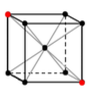Crystal Symmetry

Age 16 to 18 Challenge Level:

Use vectors and matrices to explore the symmetries of crystals.Carbon Footprints

Age 14 to 16 Challenge Level:

Is it really greener to go on the bus, or to buy local?Constantly Changing

Age 14 to 16 Challenge Level:

Many physical constants are only known to a certain accuracy. Explore the numerical error bounds in the mass of water and its constituents.Stirling Work

Age 16 to 18 Challenge Level:

See how enormously large quantities can cancel out to give a good approximation to the factorial function.Vector Walk

Age 14 to 18 Challenge Level:

Starting with two basic vector steps, which destinations can you reach on a vector walk?Fix Me or Crush Me

Age 16 to 18 Challenge Level:

Can you make matrices which will fix one lucky vector and crush another to zero?Big and Small Numbers in the Living World

Age 11 to 16 Challenge Level:

Work with numbers big and small to estimate and calculate various quantities in biological contexts.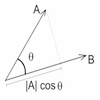Cross with the Scalar Product

Age 16 to 18 Challenge Level:

Explore the meaning of the scalar and vector cross products and see how the two are related.Scientific Curves

Age 16 to 18 Challenge Level:

Can you sketch these difficult curves, which have uses in mathematical modelling?Robot Camera

Age 14 to 16 Challenge Level:

Could nanotechnology be used to see if an artery is blocked? Or is this just science fiction?Transformations for 10

Age 16 to 18 Challenge Level:

Explore the properties of matrix transformations with these 10 stimulating questions.Square Pair

Age 16 to 18 Challenge Level:

Explore the shape of a square after it is transformed by the action of a matrix.Matrix Meaning

Age 16 to 18 Challenge Level:

Explore the meaning behind the algebra and geometry of matrices with these 10 individual problems.Nine Eigen

Age 16 to 18 Challenge Level:

Explore how matrices can fix vectors and vector directions.Pdf Stories

Age 16 to 18 Challenge Level:

Invent scenarios which would give rise to these probability density functions.Population Dynamics Collection

Age 16 to 18 Challenge Level:

This is our collection of tasks on the mathematical theme of 'Population Dynamics' for advanced students and those interested in mathematical modelling.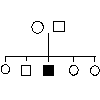Genetics

Age 14 to 16 Challenge Level:

A problem about genetics and the transmission of disease.How Do You React?

Age 14 to 16 Challenge Level:

To investigate the relationship between the distance the ruler drops and the time taken, we need to do some mathematical modelling...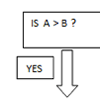Mystery Procedure

Age 14 to 16 Challenge Level:

Can you work out what this procedure is doing?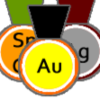Alternative Record Book

Age 14 to 18 Challenge Level:

In which Olympic event does a human travel fastest? Decide which events to include in your Alternative Record Book.What's That Graph?

Age 14 to 16 Challenge Level:

Can you work out which processes are represented by the graphs?Designing Table Mats

Age 11 to 16 Challenge Level:

Formulate and investigate a simple mathematical model for the design of a table mat.What Do Functions Do for Tiny X?

Age 16 to 18 Challenge Level:

Looking at small values of functions. Motivating the existence of the Taylor expansion.Perspective Drawing

Age 11 to 16 Challenge Level:

Explore the properties of perspective drawing.Track Design

Age 14 to 16 Challenge Level:

Where should runners start the 200m race so that they have all run the same distance by the finish?Global Warming

Age 14 to 16 Challenge Level:

How much energy has gone into warming the planet?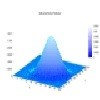Pdf Matcher

Age 16 to 18 Challenge Level:

Which pdfs match the curves?Aim High

Age 16 to 18 Challenge Level:

How do you choose your planting levels to minimise the total loss at harvest time?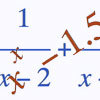Root Hunter

Age 16 to 18 Challenge Level:

In this short problem, try to find the location of the roots of some unusual functions by finding where they change sign.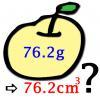More or Less?

Age 14 to 16 Challenge Level:

Are these estimates of physical quantities accurate?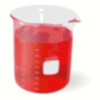Brimful 2

Age 16 to 18 Challenge Level:

Which of these infinitely deep vessels will eventually full up?Debt Race

Age 16 to 18 Challenge Level:

Who will be the first investor to pay off their debt?Reaction Rates

Age 16 to 18 Challenge Level:

Explore the possibilities for reaction rates versus concentrations with this non-linear differential equationTaking Trigonometry Series-ly

Age 16 to 18 Challenge Level:

Look at the advanced way of viewing sin and cos through their power series.Scale Invariance

Age 16 to 18 Challenge Level:

By exploring the concept of scale invariance, find the probability that a random piece of real data begins with a 1.Data Matching

Age 14 to 18 Challenge Level:

Use your skill and judgement to match the sets of random data.